# Extraction and recognition of music melody features using a deep neural network

## Zhongqing Zhang1

1Xinyang University, Xinyang, Henan, 464000, China

Journal of Vibroengineering, (in Press). https://doi.org/10.21595/jve.2023.23075
Received 25 November 2022; received in revised form 9 January 2023; accepted 18 January 2023; published 13 March 2023

Copyright © 2023 Zhongqing Zhang. This is an open access article distributed under the Creative Commons Attribution License, which permits unrestricted use, distribution, and reproduction in any medium, provided the original work is properly cited.
Views 2
CrossRef Citations 0
Abstract.

The music melody can be used to distinguish the genre style of music and can also be used for retrieving music works. This paper used a deep learning algorithm, the convolutional neural network (CNN), to extract the features of musical melodies and recognize genres. Three-tuple samples were used as training samples in the training process. Orthogonal experiments were conducted on the number of music segments and the type of activation function in the algorithm in the simulation experiments. The CNN algorithm was compared with support vector machine (SVM) and traditional CNN algorithms. The results showed that there were obvious differences in the pitch and melody curves of different genres of music; the recognition performance was best when the number of music segments was six and the activation function was relu; the CNN algorithm trained by three-tuple samples had better recognition accuracy and spent less recognition time.

Highlights
• The convolutional structure is used.
• The recognition algorithm is trained by triples.
• The number of music segments and the activation function are set using orthogonal experiments.

Keywords: music melody, pitch, feature extraction, convolutional neural network.

#### 1. Introduction

Music generally refers to the art form composed by sound, which is a sequence of phonemes arranged in a certain order and interval . With the rapid development of economy, people’s material needs are mostly satisfied, so their spiritual needs are gradually increasing. Music appreciation is a way to satisfy spiritual needs. Music as an art form has been developed over many years, and there is a market demand to satisfy spiritual needs, so many musical works and music styles exist. It is time-consuming for the average audience to retrieve the desired musical works manually . It is equally time-consuming for the learner and creator to manually analyze musical works . A melody is a component of music that corresponds to the sequence of fundamental frequency values of the pitch of the dominant tone of the music. In music analysis, a melody is also a high-level semantics of music that can be used to describe the content of a musical work. The use of musical melodies can assist in the identification and classification of musical styles and music retrieval.

Related works are as follows. Lee et al.  proposed a segment-based melody-matching method to solve the problem of wheezing noise and rhythmic inconsistency and reduce the computational complexity of the traditional linear scaling method in a singing/humming query system. The results showed that the segment-based method solved these problems better than the traditional global linear scaling method. Reddy et al.  proposed a melody extraction method based on time-domain adaptive filtering and verified the effectiveness of the method through experiments. Sunny et al.  proposed a music emotion recognition and genre classification method using a Gaussian process and a neural network. The results showed that the neural network-based Gaussian process consistently outperformed the neural network in the music emotion recognition and genre classification tasks. This paper used a deep learning algorithm, i.e., the convolutional neural network (CNN) algorithm, for feature extraction and genre recognition of musical melodies. Three-tuple samples were used as training samples. Orthogonal experiments were performed on the number of musical segments and the type of activation function in the algorithm. The CNN algorithm was compared with support vector machine (SVM) and traditional CNN algorithms.

#### 2. Extraction and recognition of music melody features

Music melody is an advanced semantic feature of music, which can reflect the content that music is intended to express to a certain extent. Using this feature is possible to achieve recognition of music style, retrieval of music, and even detection of music similarity. For ordinary listeners without professional training , although they can make intuitive judgments based on the melodies they hear, it is difficult to identify their musical styles accurately. In addition, even for professionally trained listeners, retrieval and similarity judgment of music by melodies can also take much time. In order to improve the efficiency of operations such as music style recognition and music retrieval, deep learning algorithms are applied to extract and recognize melodic features .

Fig. 1. Deep learning algorithm-based music melody feature extraction and recognition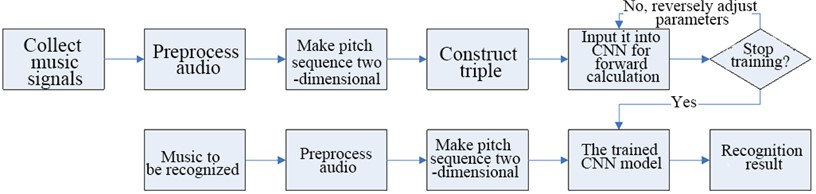The process of using a deep learning algorithm to extract and identify music melody features is shown in Fig. 1. In this paper, the authors use the pitch sequence of music signals as the feature of music melody, and the specific steps are below.

(1) The music signal dataset is collected to train the deep learning algorithm. The genre corresponding to the music is also collected as the identification label of the music signal dataset.

(2) The audio signal is preprocessed, including noise reduction and framing processing . During framing, the frame length is set as 20 ms, and the frame shift size is set as 10 ms to ensure the continuity of the fundamental frequency that can reflect the pitch.

(3) The fundamental frequency of every frame of the music signal is extracted to get the original pitch sequence. Whether the frame music signal has sound is determined based on the sound energy size. If the frame music signal has no sound, the fundamental frequency of the frame music signal is 0; if the frame music signal has sound, the Fourier transform  is performed on the frame signal to get the spectrum map. After that, the fundamental frequency of the frame signal is calculated and taken as the pitch, and the calculation formula is:

(1)
$\left\{\begin{array}{l}\begin{array}{ll}H=\left\{{f}_{i}\left|{f}_{i}={f}_{\mathrm{m}\mathrm{a}\mathrm{x}}/i\right\right\},& i=1,2,3,4,5,\end{array}\\ S\left({f}_{i}\right)=\sum _{n=1}^{M}{h}_{n}A\left(n{f}_{i}\right),\\ {f}_{0}=\mathrm{a}\mathrm{r}\mathrm{g}\mathrm{m}\mathrm{a}\mathrm{x}\left\{S\left({f}_{i}\right)\right\},\end{array}\right\$

where $H$ is the set of candidate fundamental frequencies, ${f}_{\mathrm{m}\mathrm{a}\mathrm{x}}$ is the global peak frequency in the spectrogram of the frame, $S\left({f}_{i}\right)$ is the confidence level when ${f}_{i}$ is used as the fundamental frequency, $M$ is the number of harmonics, ${h}_{n}$ is the compression factor of the $n$-th harmonic , $A\left(n{f}_{i}\right)$ is the amplitude of the $n$-th harmonic when ${f}_{i}$ is used as the fundamental frequency, and ${f}_{0}$ is the calculated fundamental frequency of the frame signal, which is the frequency with the highest confidence level among the candidate fundamental frequencies.

(4) The original pitch sequence of the music signal is the fundamental frequency of all frame music signals arranged according to time, and the original pitch sequence image of the music melody is often a stepped line. Although the CNN algorithm can also be trained to recognize the original pitch sequence image directly, the peak angle of the stepped lines will make it difficult for the CNN algorithm to learn deep features, so this paper uses the pitch histogram to extract the statistical features of the pitch sequence. The original pitch sequence is normalized. The interval of [0, 1] is divided into five equal parts. The number of pitch values in every interval is counted. Then, the statistical values are normalized. Finally, the extreme difference, variance, and mean of the normalized pitch sequence are calculated and taken as the complement of the statistical features. An eight-dimensional pitch statistical feature $\left[{c}_{1},{c}_{2},{c}_{3},{c}_{4},{c}_{5},range,\mathrm{v}\mathrm{a}\mathrm{r},mean{\right]}^{T}$ is obtained, where ${c}_{i}$ is the statistical value of the $i$-th interval after normalization, $range$ is the extreme difference of the normalized pitch sequence, $\mathrm{v}\mathrm{a}\mathrm{r}$ is the variance, and $mean$ refers to the mean value. The pitch sequence is processed to be two-dimensional, i.e., the original pitch sequence of the music signal is divided into $N$ segments, and eight-dimensional pitch statistical features are obtained from every pitch sequence according to the above method. The eight-dimensional pitch statistical features of $N$ segments are combined to obtain the two-dimensional pitch features of the music signal in a size of $8×N$.

(5) After obtaining the two-dimensional pitch features of the music signal through the above steps, they are directly used for CNN training, but in practice, the music signal used for genre style recognition cannot be completely provided by one person. Different people have different degrees of mastery of the music melody. Moreover, music segmentation during training will destroy the correlation of melodic features to some extent, so this paper trains the CNN with triple . A three-tuple sample contains an original sample, a positive sample, and a negative sample. The original sample is a music sample fragment of a genre style, the positive sample is a random music sample fragment of the same genre style as the original sample, and the negative sample is a random music sample fragment of a different genre style from the original sample.

(6) When the CNN algorithm is trained using three-tuple music samples, three CNNs with the same structure are required, one for processing the original samples, one for processing the positive samples, and one for processing the negative samples. When CNNs process the two-dimensional pitch features of music samples, they are all processed by convolutional operations in the convolutional layer using convolutional kernels :

(2)
${Y}_{i}=f\left({X}_{i}\otimes {W}_{i}+{b}_{i}\right),$

where ${Y}_{i}$ is the convolutional output feature value of the $i$-th convolutional kernel, ${X}_{i}$ is the input vector of the $i$-th convolutional kernel, ${W}_{i}$ is the weight in the $i$-th convolutional kernel, and ${b}_{i}$ is the bias of the $i$-th convolutional kernel. In addition, the convolutional features extracted by the convolutional kernels are pooled and compressed in the pooling layer in order to reduce the computational effort . After that, the computational result, which is the genre label of the music signal, is output in the fully connected layer.

(7) Whether the training of the CNN is finished is determined. If it is, the parameters in the CNN are fixed and used for testing with subsequent test sets; if it is not, the parameters in the CNN are adjusted in the reverse direction. The conditions used to judge whether the training is over include whether the number of training iterations reaches the maximum and whether the CNN objective function converges to stability. The purpose of the CNN is to identify the genre style of the music signal, so the objective function needs to reflect the difference between the identification result and the actual result. In addition, this paper also uses triple to train the CNN to make the features extracted from similar samples as close as possible and the features extracted from different samples as far away as possible. Therefore, the objective function that can reflect the feature difference between three-tuple samples is also needed. The final objective function is:

(3)
$\left\{\begin{array}{l}l={l}_{tri}+{l}_{s},\\ {l}_{s}=-{\sum }_{i=1}^{n}{\sum }_{j=1}^{c}{Z}_{ij}\mathrm{lg}\left({y}_{ij}\right),\\ {l}_{tri}=\mathrm{m}\mathrm{a}\mathrm{x}\left(0,{\sum }_{i=1}^{M}\left({‖f\left({I}_{i}\right)-f\left({I}_{i}^{+}\right)‖}_{2}^{2}-{‖f\left({I}_{i}\right)-f\left({I}_{i}^{-}\right)‖}_{2}^{2}+\alpha \right)\right),\end{array}\right\$

where $l$ is the total objective loss function, ${l}_{s}$ is the classification loss function, ${l}_{tri}$ is the triple feature expression loss function, $n$ is the total number of samples, $M$ is the total number of triples, $c$ is the number of categories, ${I}_{i}$, ${I}_{i}^{+}$, ${I}_{i}^{-}$ are the original, positive, and negative samples in the $i$-th triplet, ${Z}_{ij}$ is the decision variable that takes the value of 1 when sample $i$ actually belongs to category $j$ and 0 vice versa, ${y}_{ij}$ is the calculated probability that sample $i$ belongs to category $j$, $f\left(•\right)$ is the sample feature extracted using the CNN, $\alpha$ is the minimum interval between the original and positive sample feature spacing and the original and negative sample feature spacing.

The above steps are repeated until the total objective loss function of the CNN converges to a stable level, or the number of iterations reaches the maximum. The application process of the trained CNN is shown in Fig. 1. After processing the music segment according to steps (2), (3), and (4), the two-dimensional pitch sequence features are input into the trained CNN, and the probability distribution of the music genre style classification is obtained after forward calculation. The category with the highest probability is used as the genre style recognition result of the music fragment.

#### 3.1. Experimental data

Nottingham dataset and Theorytab were music datasets used in the experiment. The former is a classical dataset containing folk songs & ballads in the MIDI format , and the data come with chord markers. The latter has more than 10,000 western pop music songs with chord markers, containing more than 20,000 music clips. Two hundred music clips were selected from one of five genres, i.e., classical, rock, jazz, pop, and rap, in the above music datasets; 150 clips were taken as the training samples, and 50 clips were taken as the test samples. There were 1,000 music clips, among which 750 clips were the training samples and 250 were the test samples. The sampling frequency of every music clip was 44 kHz, and the duration was 30 s. The music duration selected in this paper was determined by orthogonal tests.

#### 3.2. Experimental setup

In the CNN-based music melody feature extraction and recognition algorithm, the music signal was divided into $N$ subsections when the pitch sequence was two-dimensionalized. The size of $N$ could affect the specification of the two-dimensional pitch sequence, which could affect the local features of the melody. Moreover, the type of activation function used when the convolution layer in the CNN algorithm convolved the two-dimensional pitch sequence could also affect the performance of the whole algorithm. Therefore, the orthogonal tests were performed on $N$ and activation function type. The number of $N$ was set as 2, 4, 6, 8, and 10, and the activation function type was set as relu, tahn, and sigmoid. The setting of the other relevant parameters is as follows. There were three convolutional layers, and each had 48 convolution kernels with a size of 3×3. The moving step length of the convolution kernel was 1. One pooling layer followed every convolutional layer. The moving step length of the mean-pooling box in a size of 2×2 was 1. A regularization with a random deactivation of 0.4 was used during the training process, and the maximum number of iterations was 1,500.

In order to further verify the performance of the CNN algorithm trained by three-tuple samples, it was compared with two other recognition algorithms. The first one was also a CNN algorithm, but the difference was that this CNN algorithm was not trained by three-tuple samples. The two-dimensional pitch sequence was used directly to train the CNN algorithm. The loss objective function was cross-entropy. The relevant parameters of the convolution and pooling layers were the same as the CNN algorithm trained by three-tuple samples. A regularization with a random deactivation of 0.4 was used, and the maximum number of iterations was 1,500. The second algorithm was the SVM algorithm. The statistical features of the pitch sequence were used as the input vector of the SVM algorithm. The relevant parameters of the SVM algorithms are as follows: the sigmoid function was used as the kernel function, and the penalty factor was set as 1.

#### 3.3. Evaluation indicators

(4)
$\left\{\begin{array}{l}P=\frac{TP}{TP+FP},\\ R=\frac{TP}{TP+FN},\\ F=\frac{2PR}{P+R},\end{array}\right\$

where $P$ refers to the precision, $R$ is the recall rate, $F$ is a comprehensive consideration of the precision and recall rate, $TP$ is the number of positive samples that are predicted as positive, $FP$ is the number of negative samples that are predicted as positive, and $FN$ is the number of positive samples that are predicted as negative.

#### 4. Experimental results

The number of music samples used was large, and due to space limitation, only the pitch melodic lines of part of the music clips are shown in Figs. 2-3. It was seen from the figures that the melodic pitch lines of the two different genres of music were significantly different. The characteristic points in the pitch melody lines of the classical genre were mostly scattered up and down, while the characteristic points in the pitch melodic lines of the jazz genre were relatively more concentrated.

Fig. 2. Pitch melodic lines of a music fragment belong the classical genre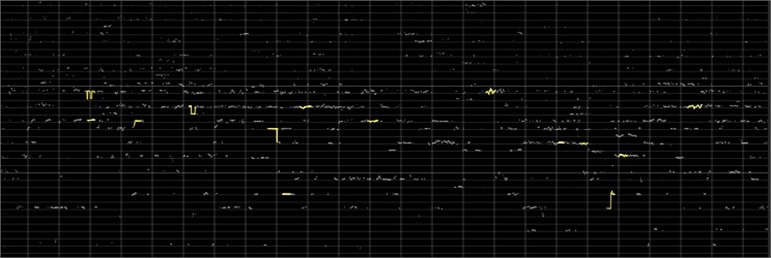The CNN algorithm trained by three-tuple samples was tested orthogonally for the number of music segments and the type of activation function, and the results are shown in Table 1. It was seen from the data in Table 1 that the performance of the CNN algorithm adopting different activation functions for music genre recognition rose first and then decreased; the CNN algorithm adopting different activation functions had the best recognition performance when the number of music segments was six; when the number of music fragments was the same, the CNN algorithm adopting the relu activation function performed the best, followed by the algorithm adopting the sigmoid activation function and the algorithm adopting the tahn activation function. After orthogonal comparison, it was concluded that the CNN algorithm trained by three-tuple samples had the best recognition performance when the number of music segments was six and the activation function was relu.

Fig. 3. Pitch melodic lines of a music fragment belonging to the jazz genre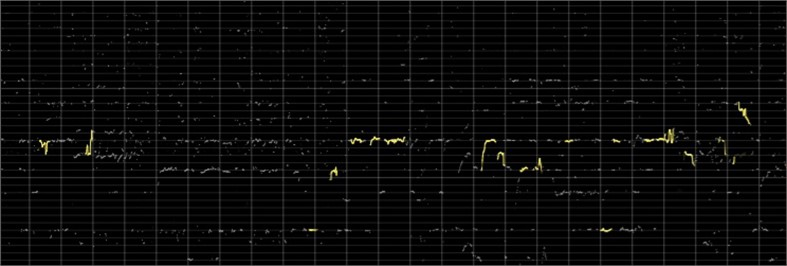Table 1. The music recognition performance of this CNN algorithm trained by the three-tuple samples under different numbers of music segments and different activation function types

 Activation function Evaluation indicators $N=2$ $N=4$ $N=6$ $N=8$ $N=10$ Relu Precision / % 81.2 85.3 89.6 85.4 80.1 Recall rate / % 82.1 85.7 87.8 85.4 81.7 F-value / % 81.6 85.5 88.7 85.4 80.9 Sigmoid Precision / % 71.5 75.7 78.9 75.4 71.2 Recall rate / % 71.2 74.3 77.8 73.7 70.2 F-value / % 71.3 75.0 78.3 74.5 70.7 Tahn Precision / % 69.8 72.1 75.6 72.3 70.1 Recall rate / % 69.7 71.8 74.8 71.3 70.2 F-value / % 69.7 71.9 75.2 71.8 70.1

After obtaining the appropriate number of music segments and the type of the activation function through orthogonal experiments, the performance of the CNN algorithm trained by three-tuple samples was compared with two other algorithms, SVM and traditional CNN algorithms, in order to further verify its performance in recognizing music genres. The SVM algorithm used the six-dimensional statistical features of the pitch sequence directly when recognizing music genres, while the traditional CNN algorithm divided the music into different parts and transformed them into two-dimensional pitch sequence statistical features. The test results of the performance of the three recognition algorithms are shown in Fig. 4. The precision, recall rate, and F-value of the SVM algorithm were 72.3 %, 71.8 %, and 72.0 %, respectively; the precision, recall rate, and F-value of the traditional CNN algorithm were 82.5 %, 82.1 %, and 82.3 %, respectively; the precision, recall rate, and F-value of the CNN algorithm trained by three-tuple samples were 89.6 %, 87.8 %, and 88.7 %, respectively. It was found from the comparison in Fig. 4 that the CNN algorithm trained by three-tuple samples had the best performance, the traditional CNN algorithm was the second, and the SVM algorithm was the worst.

In addition to comparing the detection accuracy of the three music genre recognition algorithms, the average time spent on genre recognition of music fragments was also compared between the three algorithms, and the results are shown in Fig. 5. The average time spent on recognition of every music fragment by the SVM algorithm was 2.42 s, the average time of the traditional CNN algorithm was 1.32 s, and the average time of the CNN algorithm trained by three-tuple samples was 1.24 s. It was seen from Fig. 5 that the SVM algorithm took the longest time to recognize music clips, followed by the traditional CNN and the CNN algorithm trained by three-tuple samples.

Fig. 4. Performance of three music genre recognition algorithms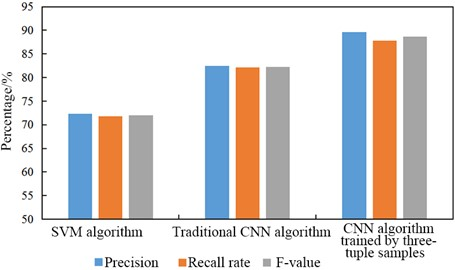Fig. 5. Average recognition time of three music genre recognition algorithms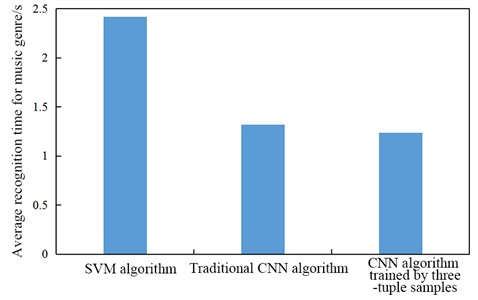#### 5. Discussion

The music melody is an advanced semantic meaning of a musical work, corresponding to the sequence of fundamental frequency values of pitches in the dominant music tone. Melody can be used to describe what the music is trying to say. Analyzing musical melodies can help understand the genre of music and can be used to search for musical works to aid in the appreciation and creation of music. However, as different people have different music appreciation abilities and different music-related professional literacy and the number of music works is large, it is difficult for the general public to judge music styles or retrieve related works by virtue of melodies. In this paper, deep learning was used to extract and recognize the features of music melodies. Three-tuple samples were used to train the CNN algorithm to improve its recognition performance, and the objective functions of the difference between original and positive sample features and the difference between original and negative sample features were added to guide the learning direction of the algorithm. Finally, the number of music segments and the activation function type in the CNN algorithm trained by three-tuple samples were orthogonalized in the simulation experiment, and it was compared with SVM and traditional CNN algorithms. The results have been shown in the previous section.

The results of the orthogonal experiments showed that the CNN algorithm trained by three-tuple samples had the best recognition performance when the number of music segments was six and the activation function was relu. The reason is as follows. In order to reduce the influence of the peak angle of the stepped lines of the pitch sequence, the statistical features of the pitch sequence were used when constructing the pitch sequence features. After segmenting the music, the statistical features contained both global and local features. The increase of segments increased the local features, so the accuracy increased, but when there were too many segments, local features were lost, so the accuracy decreased. Regarding activation functions, tahn and sigmoid have little variation with the input quantity at the edges and are easy to fall into locally optimal solutions in the training process, so the algorithm adopting the relu activation function performed the best. In addition, in the orthogonal experiments, the recognition performance of the CNN algorithm under different activation functions tended to increase first and then decrease with the increase of the number of music segments. The reason for this result is as follows. The segmentation of music increased the local music features, and the combination of segmented music generated global features, so the segmentation could improve the recognition performance. The increase in the number of segments meant an increase in the number of local features, so the recognition performance strengthened initially, but when the number of segments was too much, the length of segmented music was insufficient to bear enough features, which led to the loss of features and eventually made the recognition performance decline.

The comparison of the three recognition algorithms showed that the CNN algorithm trained by three-tuple samples had the best recognition accuracy and took the least time to recognize, the traditional CNN algorithm was the second, and the SVM algorithm was the worst. The reason is as follows. The SVM algorithm directly adopted the statistical features of pitch sequence to recognize the genres of music fragments and did not profoundly analyze the connection between the features. When recognizing the genres of music fragments, the traditional CNN algorithm obtained the two-dimensional statistical features of the pitch sequence by segmentation and recombination, which takes into account the global and local features. Then, the deep connection between the features was mined through the convolution layer of the CNN. Thus, its accuracy was higher. The CNN algorithm proposed in this paper used three-tuple samples for training. In the training process, the actual results were compared with the calculated results, and the original and positive sample feature differences and the original and negative sample feature differences were also compared. Thus, it had the highest recognition accuracy.

#### 6. Conclusions

This paper used the CNN algorithm to extract features from music melodies and recognize genres, took three-tuple samples as training samples, carried out orthogonal experiments on the number of music segments and the type of activation function, and compared the CNN algorithm trained by three-tuple samples with SVM and traditional CNN algorithms. The results are shown below. There were significant differences in the pitch melodic lines of different genres of music. When the number of music segments was six and the activation function was relu, the CNN algorithm trained by three-tuple samples had the best recognition performance. The CNN algorithm trained by three-tuple samples had the best recognition accuracy, followed by the traditional CNN algorithm and the SVM algorithm. The SVM algorithm took the longest average recognition time, the traditional CNN algorithm was the second, and the CNN algorithm trained by three-tuple samples took the shortest time.

#### Acknowledgements

The authors have not disclosed any funding.

1. S. A. Herff, K. N. Olsen, and R. T. Dean, “Resilient memory for melodies: The number of intervening melodies does not influence novel melody recognition,” Quarterly Journal of Experimental Psychology, Vol. 71, No. 5, pp. 1150–1171, May 2018, https://doi.org/10.1080/17470218.2017.1318932 [Publisher]
2. M. Bomgardner, “MATERIALS Schlumberger pilots new lithium extraction,” Chemical and Engineering News: “News Edition” of the American Chemical Society, Vol. 99, No. 11, 2021. [Search CrossRef]
3. Yanfang Wang and Yanfang Wang, “Research on handwritten note recognition in digital music classroom based on deep learning,” Journal of Internet Technology, Vol. 22, No. 6, pp. 1443–1455, Nov. 2021, https://doi.org/10.53106/160792642021112206020 [Publisher]
4. Wen-Hsing Lai and Chi-Yong Lee, “Query by singing / humming system using segment-based melody matching for music retrieval,” WSEAS Transactions on Systems, Vol. 15, pp. 157–167, 2016. [Search CrossRef]
5. M. Gurunath Reddy and K. Sreenivasa Rao, “Predominant melody extraction from vocal polyphonic music signal by time-domain adaptive filtering-based method,” Circuits, Systems, and Signal Processing, Vol. 37, No. 7, pp. 2911–2933, Jul. 2018, https://doi.org/10.1007/s00034-017-0696-1 [Publisher]
6. F. Sunny, V. Ssreevarsha, K. Jamseera, and P. Nijisha, “Music genre and emotion recognition using gaussian processes and neural network,” International Journal of Advance Research and Innovative Ideas in Education, Vol. 3, pp. 1020–1022, 2014. [Search CrossRef]
7. A. Paul, R. Pramanik, S. Malakar, and R. Sarkar, “An ensemble of deep transfer learning models for handwritten music symbol recognition,” Neural Computing and Applications, Vol. 34, No. 13, pp. 10409–10427, Jul. 2022, https://doi.org/10.1007/s00521-021-06629-9 [Publisher]
8. P. Hoffmann and B. Kostek, “Bass enhancement settings in portable devices based on music genre recognition,” Journal of the Audio Engineering Society, Vol. 63, No. 12, pp. 980–989, Jan. 2016, https://doi.org/10.17743/jaes.2015.0087 [Publisher]
9. X. Wang, “Research on the improved method of fundamental frequency extraction for music automatic recognition of piano music,” Journal of Intelligent and Fuzzy Systems, Vol. 35, No. 3, pp. 2777–2783, Oct. 2018, https://doi.org/10.3233/jifs-169630 [Publisher]
10. Z. Xiao, X. Chen, and L. Zhou, “Real-time optical music recognition system for dulcimer musical robot,” Journal of Advanced Computational Intelligence and Intelligent Informatics, Vol. 23, No. 4, pp. 782–790, Jul. 2019, https://doi.org/10.20965/jaciii.2019.p0782 [Publisher]
11. D. F. Silva, C.-C. M. Yeh, Y. Zhu, G. E. A. P. A. Batista, and E. Keogh, “Fast similarity matrix profile for music analysis and exploration,” IEEE Transactions on Multimedia, Vol. 21, No. 1, pp. 29–38, Jan. 2019, https://doi.org/10.1109/tmm.2018.2849563 [Publisher]
12. M. Schwabe and M. Heizmann, “Influence of input data representations for time-dependent instrument recognition,” tm – Technisches Messen, Vol. 88, No. 5, pp. 274–281, May 2021, https://doi.org/10.1515/teme-2020-0100 [Publisher]
13. G. Fernández-Rubio, F. Carlomagno, P. Vuust, M. L. Kringelbach, and L. Bonetti, “Associations between abstract working memory abilities and brain activity underlying long-term recognition of auditory sequences,” PNAS Nexus, Vol. 1, No. 4, pp. 1–10, Sep. 2022, https://doi.org/10.1093/pnasnexus/pgac216 [Publisher]
14. K. S. Gupta, “Development of music player application using emotion recognition,” International Journal for Modern Trends in Science and Technology, Vol. 7, No. 1, pp. 54–57, 2021. [Publisher]
15. P. Patil, S. Mengade, P. Kolpe, V. Gawande, and K. Budhe, “Song search engine based on querying by singing/humming,” International Journal for Scientific Research and Development, Vol. 3, No. 1, pp. 14–16, 2015. [Search CrossRef]